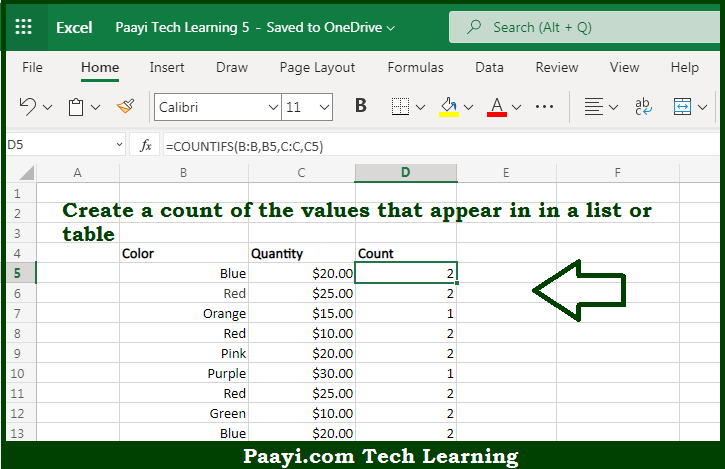# Learn How to COUNT Items in a List in Microsoft Excel

Written by | 0 Comments | 433 Views

In this article, you will learn how to COUNT various things in Microsoft Excel using a single or combination of functions and its purpose in Microsoft Excel. You will also get to know how to Count Items in a List and see the generic formula.

Count Items in a List in Microsoft Excel

The main purpose of this formula is to count the number of items that appear in the given list. Here we will learn how to create a count of the values that appear in a list or table in Microsoft Excel. That implies, with the help of a formula based on the COUNTIFS function you can able to create a count of the values that appear in a list or table. So, with the help of this formula, you can able to count the number of items that appear in the given list.

General Formula to Count Items in a List

=COUNTIFS(range1,critera1,range2,critera2)

The Explanation for the Count Items in a ListSo we know that with the help of the given formula above you can able to count the number of items that appear in the given list. Here we will learn how to create a count of the values that appear in a list or table in Microsoft Excel. As we know that, the COUNTIFS function takes multiple criteria in pairs, which means each pair contains one range and the associated criteria for that range. To give the result of a count, all conditions must match. It should be noted that to add more conditions, just add another range/criteria pair. So, with the help of this formula, you can able to count the number of items that appear in the given list.